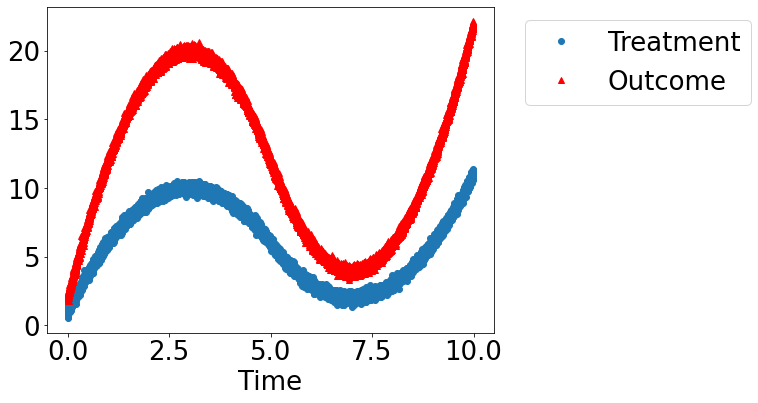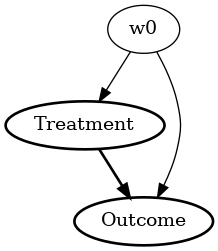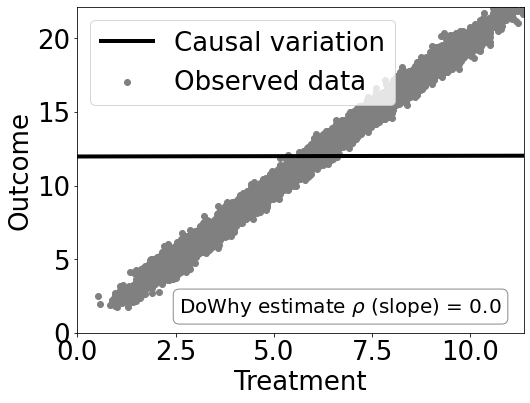# Confounding Example: Finding causal effects from observed data

Suppose you are given some data with treatment and outcome. Can you determine whether the treatment causes the outcome, or the correlation is purely due to another common cause?

:

import numpy as np
import pandas as pd
import matplotlib.pyplot as plt
import math
import dowhy
from dowhy import CausalModel
import dowhy.datasets, dowhy.plotter

# Config dict to set the logging level
import logging.config
DEFAULT_LOGGING = {
'version': 1,
'disable_existing_loggers': False,
'loggers': {
'': {
'level': 'INFO',
},
}
}

logging.config.dictConfig(DEFAULT_LOGGING)


## Let’s create a mystery dataset for which we need to determine whether there is a causal effect.

Creating the dataset. It is generated from either one of two models: * Model 1: Treatment does cause outcome. * Model 2: Treatment does not cause outcome. All observed correlation is due to a common cause.

:

rvar = 1 if np.random.uniform() >0.5 else 0
data_dict = dowhy.datasets.xy_dataset(10000, effect=rvar,
num_common_causes=1,
sd_error=0.2)
df = data_dict['df']

   Treatment    Outcome        w0
0  10.362613  21.104370  4.505220
1   2.263830   4.294143 -3.798739
2   2.626503   4.822208 -3.550164
3   8.196241  16.881973  2.401275
4   3.094419   6.385033 -2.857286

:

dowhy.plotter.plot_treatment_outcome(df[data_dict["treatment_name"]], df[data_dict["outcome_name"]],
df[data_dict["time_val"]])## Using DoWhy to resolve the mystery: Does Treatment cause Outcome?

### STEP 1: Model the problem as a causal graph

Initializing the causal model.

:

model= CausalModel(
data=df,
treatment=data_dict["treatment_name"],
outcome=data_dict["outcome_name"],
common_causes=data_dict["common_causes_names"],
instruments=data_dict["instrument_names"])
model.view_model(layout="dot")


Showing the causal model stored in the local file “causal_model.png”

:

from IPython.display import Image, display
display(Image(filename="causal_model.png"))### STEP 2: Identify causal effect using properties of the formal causal graph

Identify the causal effect using properties of the causal graph.

:

identified_estimand = model.identify_effect(proceed_when_unidentifiable=True)
print(identified_estimand)

Estimand type: nonparametric-ate

### Estimand : 1
Estimand name: backdoor
Estimand expression:
d
────────────(Expectation(Outcome|w0))
d[Treatment]
Estimand assumption 1, Unconfoundedness: If U→{Treatment} and U→Outcome then P(Outcome|Treatment,w0,U) = P(Outcome|Treatment,w0)

### Estimand : 2
Estimand name: iv
No such variable found!

### Estimand : 3
Estimand name: frontdoor
No such variable found!



### STEP 3: Estimate the causal effect

Once we have identified the estimand, we can use any statistical method to estimate the causal effect.

Let’s use Linear Regression for simplicity.

:

estimate = model.estimate_effect(identified_estimand,
method_name="backdoor.linear_regression")
print("Causal Estimate is " + str(estimate.value))

# Plot Slope of line between treamtent and outcome =causal effect
dowhy.plotter.plot_causal_effect(estimate, df[data_dict["treatment_name"]], df[data_dict["outcome_name"]])

Causal Estimate is 0.00469169526948221### Checking if the estimate is correct

:

print("DoWhy estimate is " + str(estimate.value))
print ("Actual true causal effect was {0}".format(rvar))

DoWhy estimate is 0.00469169526948221
Actual true causal effect was 0


### Step 4: Refuting the estimate

We can also refute the estimate to check its robustness to assumptions (aka sensitivity analysis, but on steroids).

### Adding a random common cause variable

:

res_random=model.refute_estimate(identified_estimand, estimate, method_name="random_common_cause")
print(res_random)

Refute: Add a Random Common Cause
Estimated effect:0.00469169526948221
New effect:0.004663014187681114



### Replacing treatment with a random (placebo) variable

:

res_placebo=model.refute_estimate(identified_estimand, estimate,
method_name="placebo_treatment_refuter", placebo_type="permute")
print(res_placebo)

Refute: Use a Placebo Treatment
Estimated effect:0.00469169526948221
New effect:-7.444932894999923e-05
p value:0.47



### Removing a random subset of the data

:

res_subset=model.refute_estimate(identified_estimand, estimate,
method_name="data_subset_refuter", subset_fraction=0.9)
print(res_subset)

Refute: Use a subset of data
Estimated effect:0.00469169526948221
New effect:0.004670042418977669
p value:0.45



As you can see, our causal estimator is robust to simple refutations.